How to use the
Learning Portal

## Learning Portal

Our hands-on, comprehensive lesson plans span a range of levels. Browse our free STEM and coding learning resources.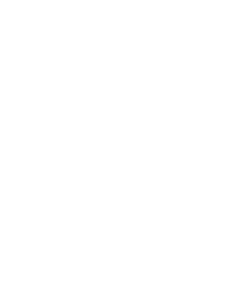# Check Learning - Variables

## What you will do:

Show what you remember about variables.

## What you need to show:

What a variables is.

How variables are used.

How variables can change.

## Vocabulary:

Variable - a named value that can be changed.

## Remember this:

Variables need to be created before they can be used and variables are often given a value when they are created.

Here is a program from MartyBlocks. Which video shows how the code instructs Marty to act? Click under the one that you think is correct.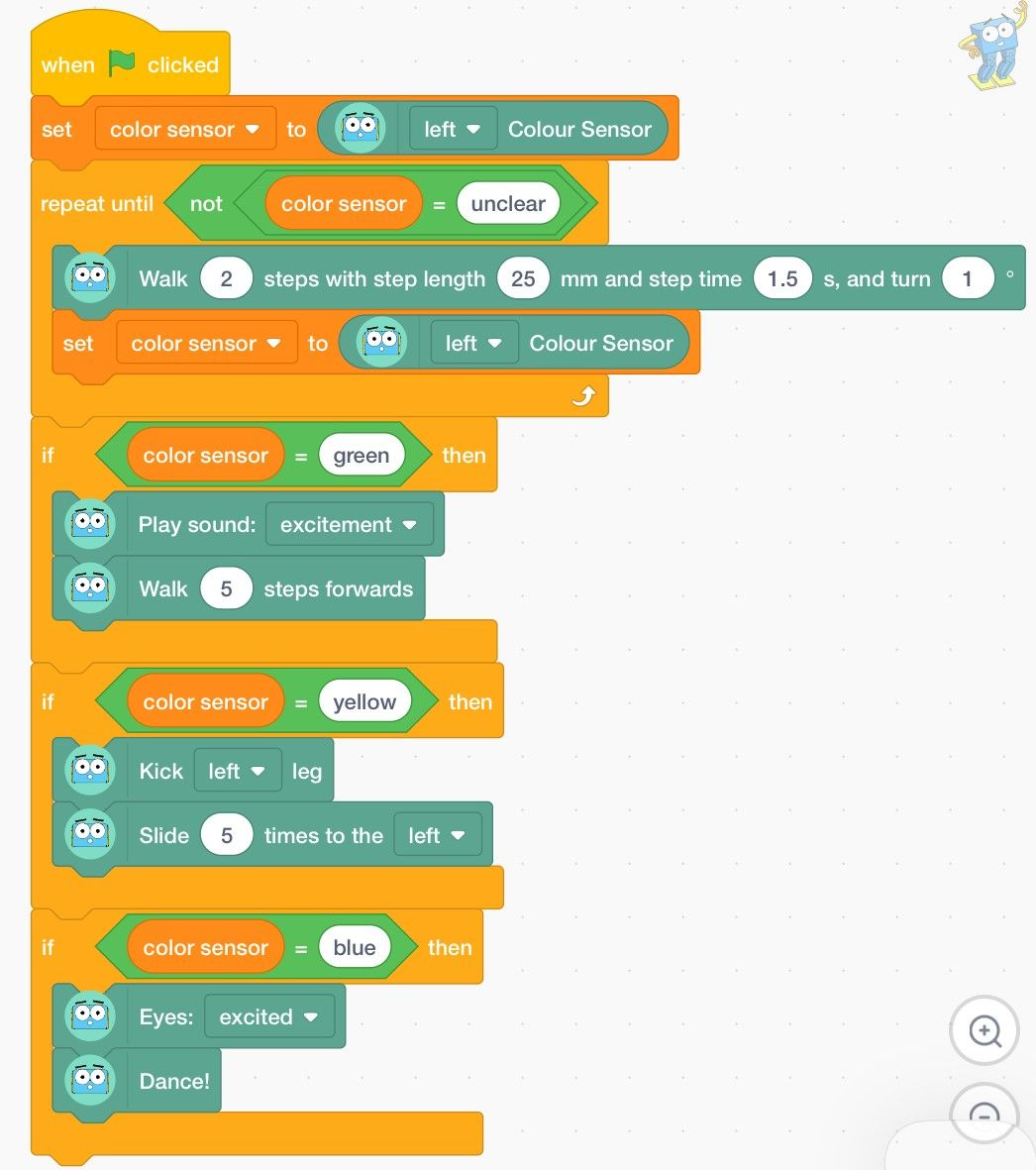Is this the one? In this video Marty kicks and sidesteps when the variable is green. That's not what is in the code. Try again. Is this the one? In this video, Marty dances when the variable is blue. You got it right, well done! Is this the one? In this video Marty makes a noise and walks forward when the variable is yellow. The code suggests that is what should happen when green is detected. Give it another try.

Click to watch a video of Marty performing several actions then select the block of code you think is correct for the actions Marty completes.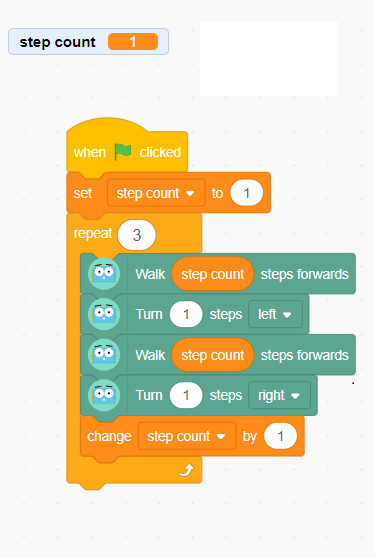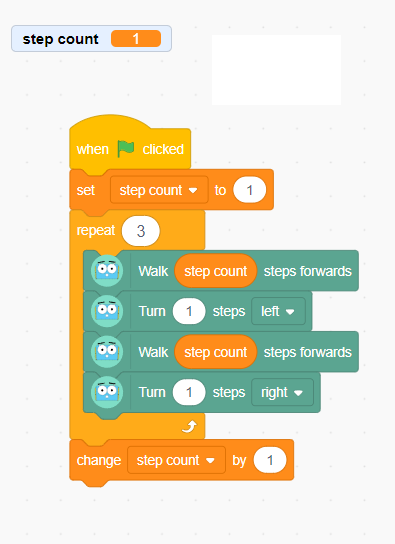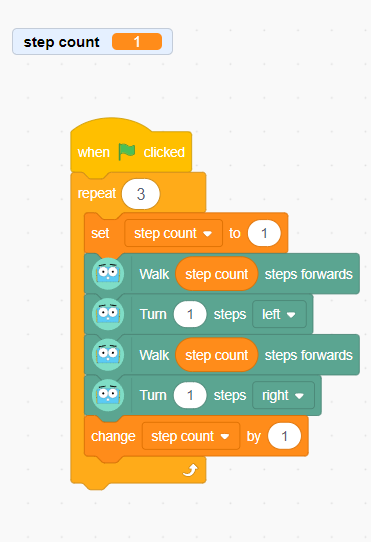Is this the one? This code sets the step count variable to one and then after each loop, the variable increases by one, so Marty takes one more steps when moving forward. This is what Marty does in the video, so well done! Is this the one? This code sets the step count variable to one and then Marty takes one step forward and turns with each loop. The variable value doesn't change until after the loop is finished. Nice try, but not quite. Is this the one? This code sets the step count variable to one and then after each loop, the variable increases by one, but then at the start of each loop, the variable is changed back to 1. So Marty will only ever take one step at a time, when moving forward. Good effort, but not quite.

There are three variables used in this block of code. Click the option you think shows the correct value for each of the variables at the end of the program.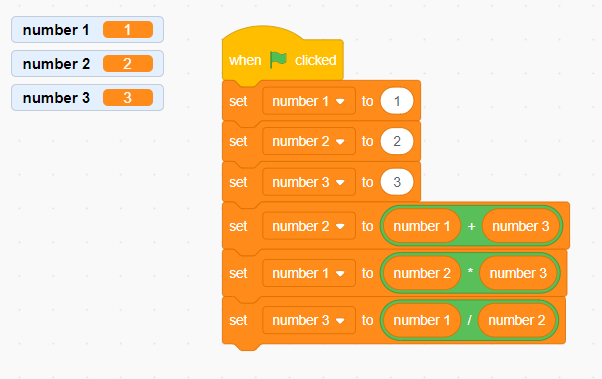Number 1 is equal to 1. Number 2 is equal to 2. Number3 is equal to 3. Is this the one? These values are correct up to the fourth block of the code but then they change. Look again to see the amounts. Number 1 is equal to 5. Number 2 is equal to 15. Number3 is equal to 1/3. Is this the one? You need to be careful with the order of the variables. You might have mixed up variables 1 and 2. Number 1 is equal to 12. Number 2 is equal to 4. Number3 is equal to 3. Is this the one? This is exactly correct! Well done seeing which variable to use and well done with the math!.

## Feedback (Here are some questions to think about):

Did you remember to that a variable needs a value before you start using it?

Did you remember that variable values can change?

Did you remember that variables don't have to be numbers?